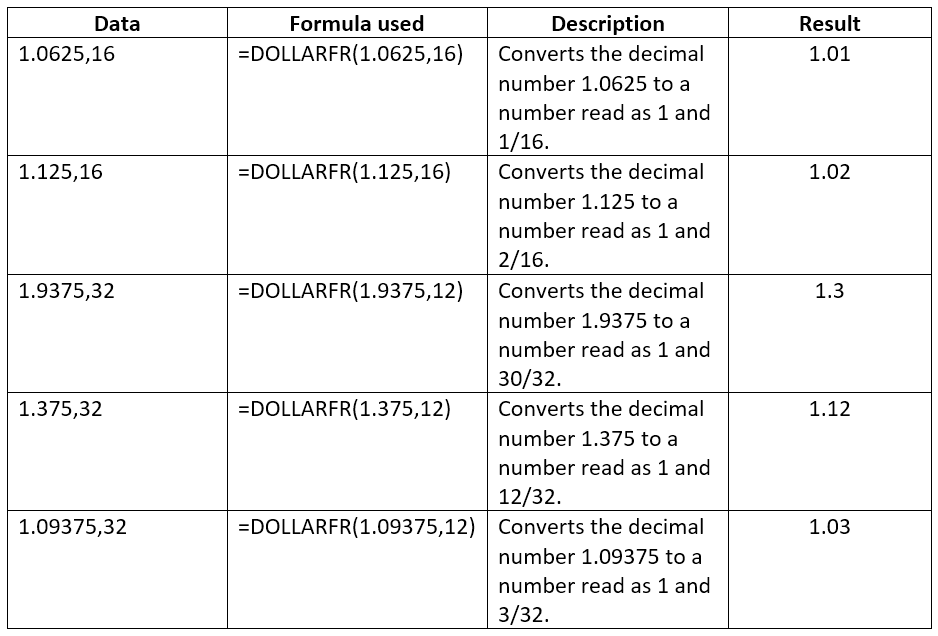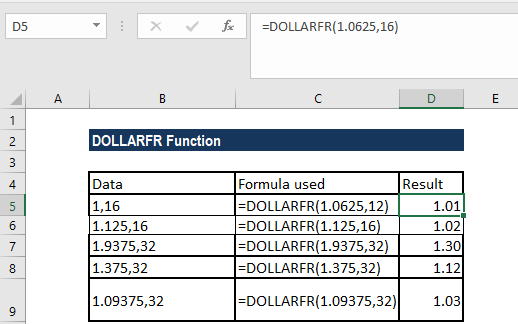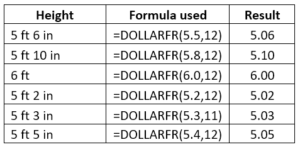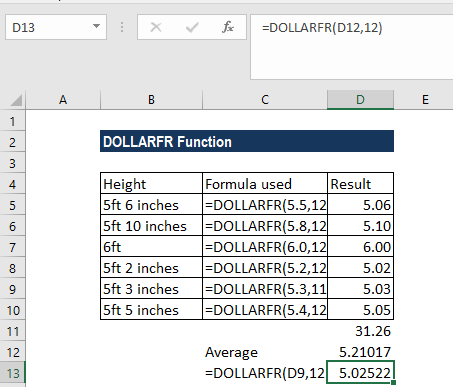# DOLLARFR Function

Converts a dollar value in decimal notation into a dollar value in fractional notation

## What is the DOLLARFR Function?

The DOLLARFR function is an Excel Financial function. DOLLARFR helps in converting a dollar value in decimal notation into a dollar value expressed in fractional notation. The function was introduced in MS Excel 2007.

DOLLARFR is very useful when dealing with financial reports and stock market quotes. Financial analysts often see prices as 1 4/16 or in the form of 1.25; the function helps them convert the figures.

### Formula

=DOLLARFR(decimal_dollar, fraction)

The DOLLARFR function uses the following arguments:

1. Decimal_dollar (required argument) – This is the dollar value expressed as a decimal number.
2. Fraction (required argument) – This is the integer to be used in the denominator of a fraction. We need to provide it as an integer but if the decimal value is provided, MS Excel will truncate it to an integer.

The function converts the decimal part of the “decimal_dollar” number into a fraction with the denominator being “fraction.”

### How to use the DOLLARFR Function in Excel?

To understand the uses of the DOLLARFR function, let’s consider a few examples:

#### Example 1

Let’s see how the function works using the examples shown below:As seen above, the DOLLARFR() function takes two arguments: the number and an integer for the denominator.

The results in Excel are shown below:#### Example 2

The DOLLARFR function is also useful to convert non-dollar data. For example, we are given the height of several students and we wish to convert them into decimal. It can be done, as shown below:Suppose we sum up the results, we will get the result as 31.26 and can find out the average as 5.21. If we wish to know the average in feet and inches, we can apply the DOLLARFR function. The result we will get is 5 feet 3 inches.### Few notes about the DOLLARFR Function

1. If the fraction given is not an integer, it will be truncated.
2. #NUM! error – Occurs when the fraction is less than zero.
3. #DIV/0 error – Occurs when the fraction given is zero.
4. #VALUE! error – This occurs if any of the given argument is non-numeric.
5. The DOLLARFR function is for display only. We can’t use the value it returns in other calculations or in charts. If we wish to do calculations on such values, we will need to reconvert them into decimal values using the DOLLARDE() function.
6. The function accepts a reference to a cell so we can provide the arguments in a different cell and refer to them to the DOLLARFR formula.
7. For users with MS Excel 2003, remember that DOLLARFR function is only available when we the Analysis ToolPak add-in is loaded.

Thanks for reading CFI’s guide to important Excel functions! By taking the time to learn and master these functions, you’ll significantly speed up your financial analysis. To learn more, check out these additional CFI resources:

• Excel Functions for Finance